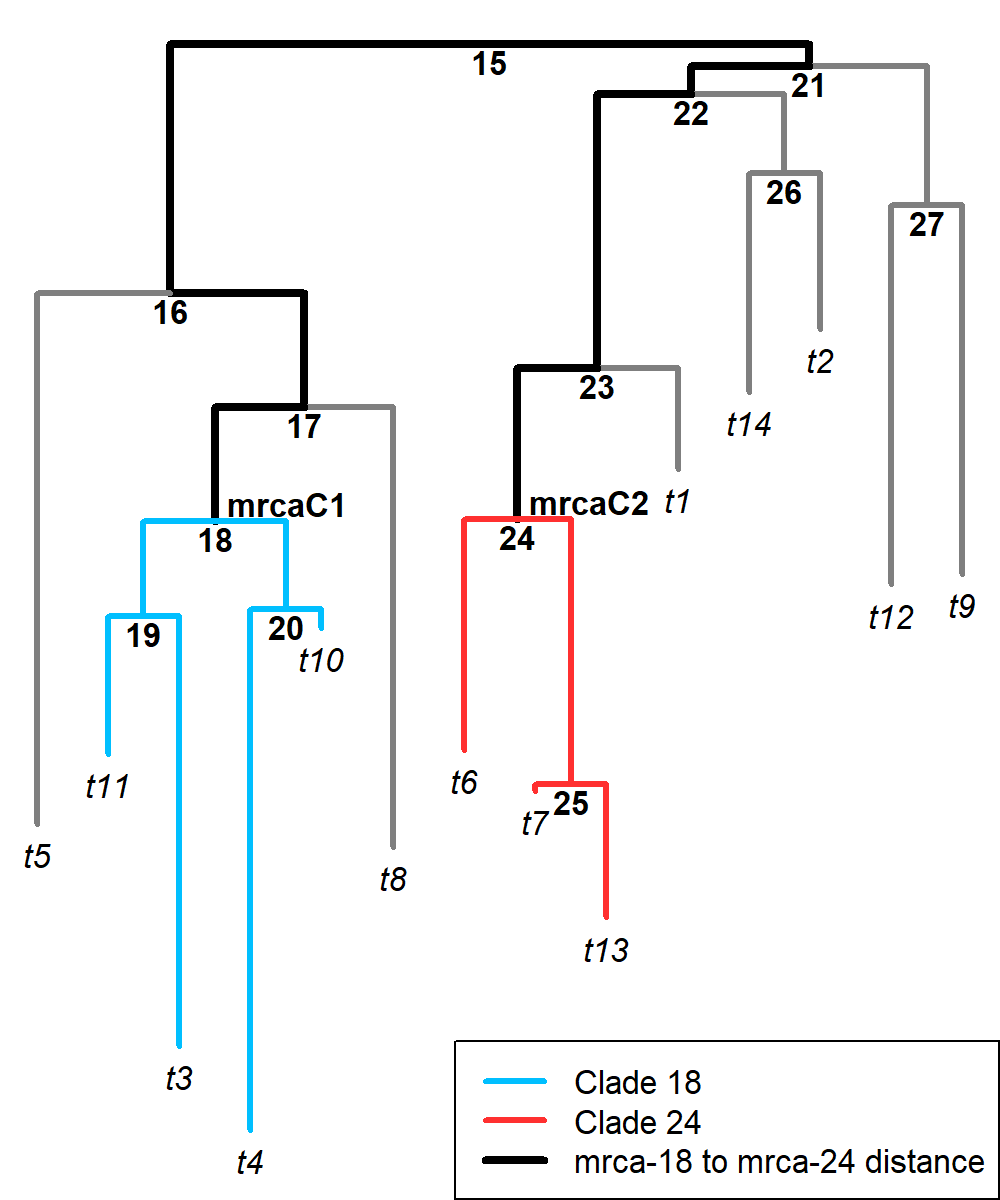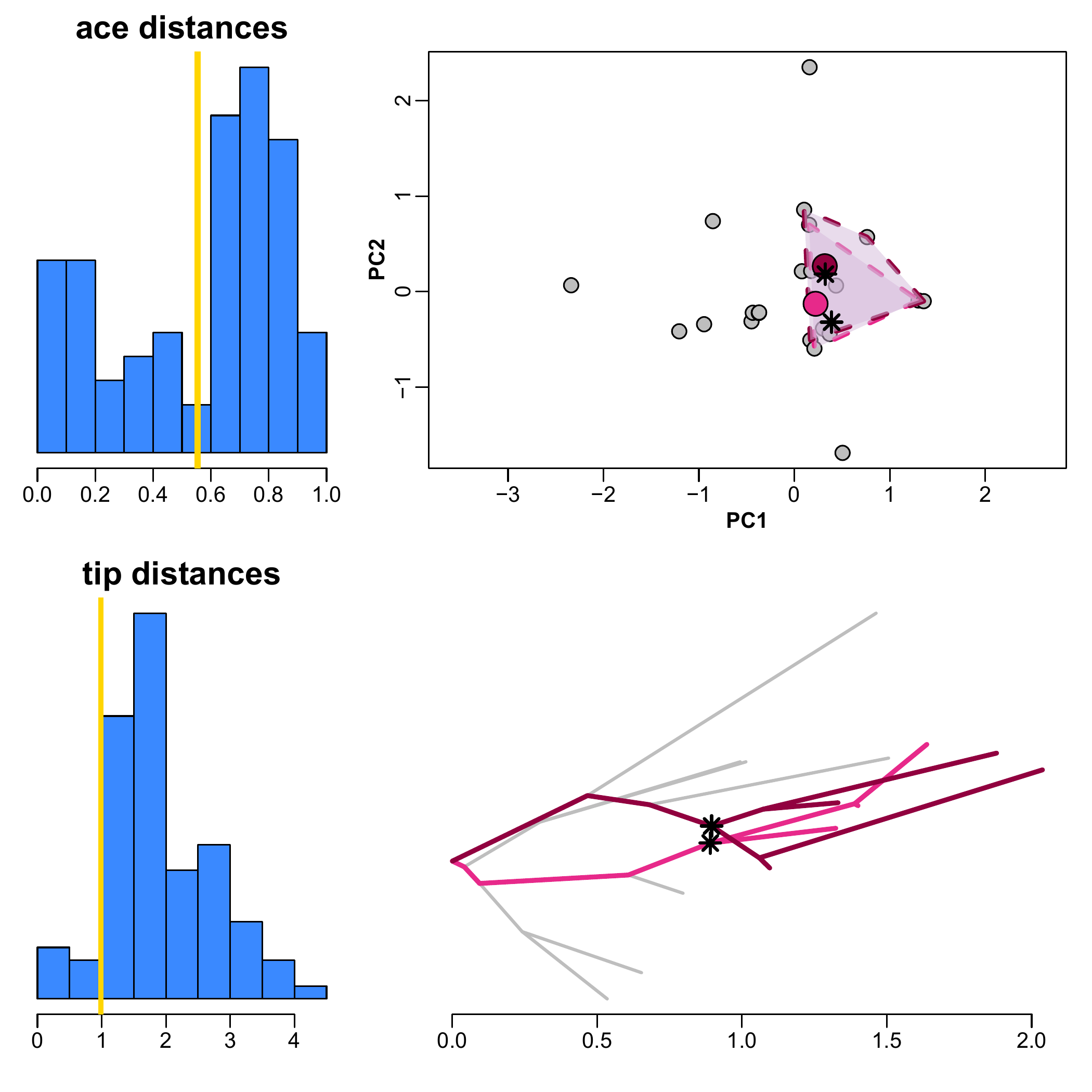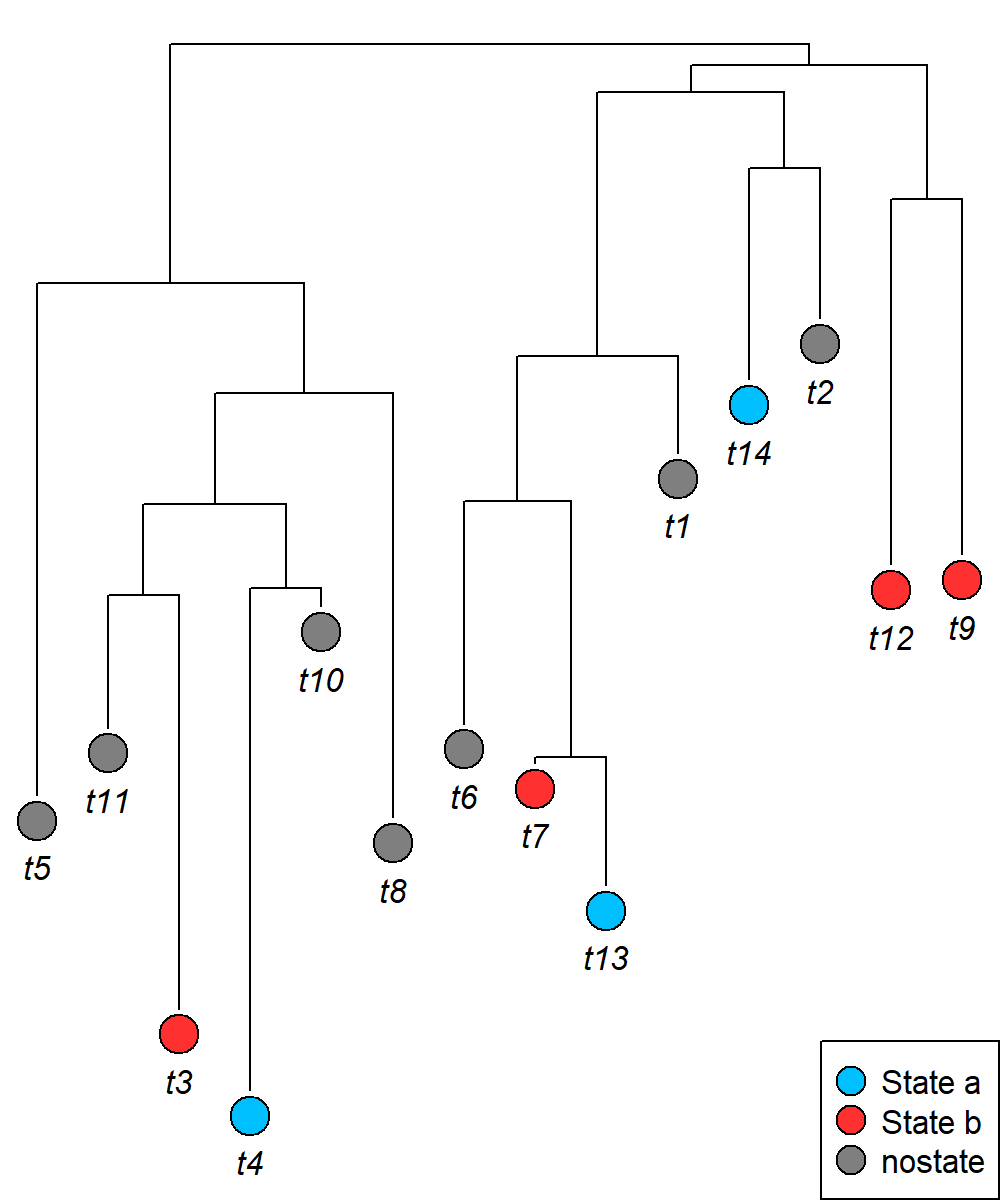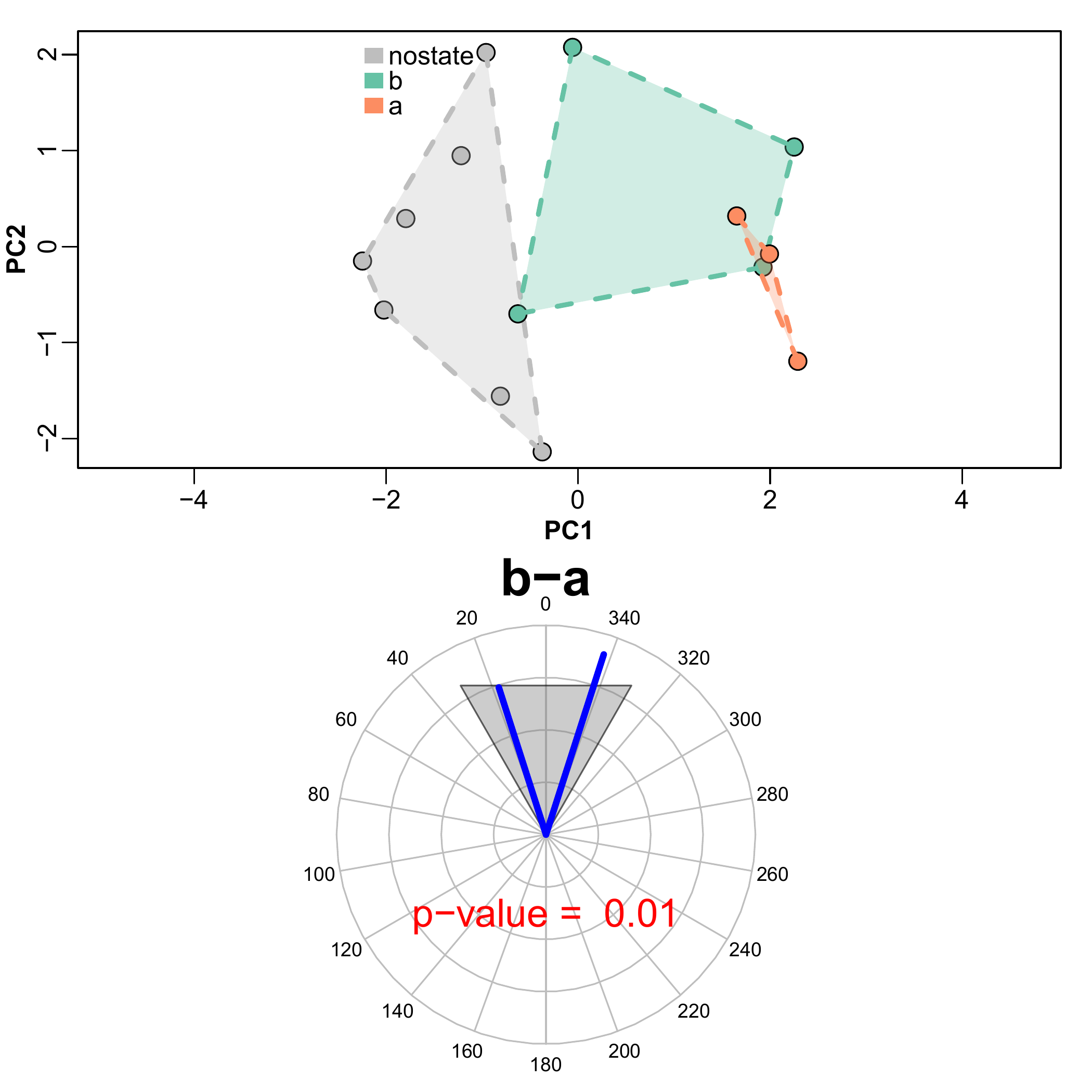# Searching for morphological convergence

## search.conv basics

Dealing with multivariate data, each species at the tree tips is represented by a phenotypic vector, including one entry value for each variable. Naming $$A$$ and $$B$$ the phenotypic vectors of a given pair of species in the tree, the angle $$θ$$ between them is computed as the inverse cosine of the ratio between the dot product of $$A$$ and $$B$$, and the product of vectors sizes: $θ = arccos(\frac{A•B}{|A||B|})$ The cosine of angle $$θ$$ actually represents the correlation coefficient between the two vectors. As such, it exemplifies a measure of phenotypic resemblance. Possible $$θ$$ values span from 0° to 180°. Small angles (i.e. close to 0˚) imply similar phenotypes. At around 90˚ the phenotypes are dissimilar, whereas towards 180˚ the two phenotypic vectors point in opposing directions (i.e. the two phenotypes have contrasting values for each variable). For a phenotype with $$n$$ variables, the two vectors intersect at a vector of $$n$$ zeros.

However, it is important to note that with geometric morphometric data (PC scores) the origin coincides with the consensus shape (where all PC scores are 0), so that, for instance, a large $$θ$$ indicates the two species diverge from the consensus in opposite directions and the phenotypic vectors can be visualized in the PC space (see the figures below).

Under the Brownian Motion (BM) model of evolution, the phenotypic dissimilarity between any two species in the tree (hence the $$θ$$ angle between them) is expected to grow proportionally to their phylogenetic distance. In the figure above, the mean directions of phenotypic change from the consensus shape formed by the species in two distinct clades (in light colors) diverge by a large angle (represented by the blue arc). This angle is expected to be larger than the angle formed by the direction of phenotypic change calculated at the ancestors of the two clades (the red arc).

Under convergence, the expected positive relationship between phylogenetic and phenotypic distances is violated and the mean angle between the species of the two clades will be shallow.

One particular case of convergence applies when species in the two clades start from similar ancestral phenotypes and tend to remain similar, on average, despite the passing of evolutionary time. These parallel trajectories are evident in the figure above, representing two clades evolving towards the same mean phenotype.

The function search.conv (Castiglione et al. 2019) is specifically meant to calculate $$θ$$ values and to test whether actual $$θ$$s between groups of species are smaller than expected by their phylogenetic distance. The function tests for convergence in either entire clades or species grouped under different evolutionary ‘states’.

When convergence between clades is tested, the user indicates the clade pair supposed to converge by setting the argument node. Otherwise, the function automatically scans the phylogeny searching for significant instance of convergent clades. In this case, the minimum distance (meant as either number of nodes or evolutionary time), and the maximum and minimum sizes (in term of number of tips) for the clades to be tested are pre-set within the function or indicated by the user through the arguments min.dist, max.dim, and min.dim, respectively.

Given two monophyletic clades (subtrees) $$C1$$ and $$C2$$, search.conv computes the mean angle $$θ_{real}$$ over all possible combinations of pairs of species taking one species per clade. This $$θ_{real}$$ is divided by the patristic (i.e. the sum of branch lengths) distance between the most recent common ancestors (mrcas) to $$C1$$ and $$C2$$, $$mrcaC1$$ and $$mrcaC2$$, respectively, to account for the fact that the mean angle (hence the phenotypic distance) is expected to increase, on average, with phylogenetic distance. To assess significance, search.conv randomly takes a pair of tips from the tree ($$t1$$ and $$t2$$), computes the angle $$θ_{random}$$ between their phenotypes and divides $$θ_{random}$$ by the distance between $$t1$$ and $$t2$$ respective immediate ancestors (i.e. the distance between the first node $$N1$$ above $$t1$$, and the first node $$N2$$ above $$t2$$). This procedure is repeated 1,000 times generating $$θ_{random}$$ per unit time values, directly from the tree and data. The $$θ_{random}$$ per unit time distribution is used to test whether $$θ_{real}$$ divided by the distance between $$mrcaC1$$ and $$mrcaC2$$ is statistically significant, meaning if it is smaller than 5% of $$θ_{random}$$ values the two clades are said to converge.

With seach.conv, it is also possible to test for the initiation of convergence. In fact, given a pair of candidate clades under testing, the phenotypes at $$mrcaC1$$ and $$mrcaC2$$ are estimated by RRphylo, and the angle between the ancestral states ($$θ_{ace}$$) is calculated. Then, $$θ_{ace}$$ is added to $$θ_{real}$$ and the resulting sum divided by the distance between $$mrcaC1$$ and $$mrcaC2$$. The sum $$θ_{ace} + θ_{real}$$ should be small for clades evolving from similar ancestors towards similar daughter phenotypes. Importantly, a small $$θ_{ace}$$ means similar phenotypes at the mrcas of the two clades, whereas a small $$θ_{real}$$ implies similar phenotypes between their descendants. It does not mean, though, that the mrcas have to be similar to their own descendants. Two clades might, in principle, start with certain phenotypes and both evolve towards a similar phenotype which is different from the initial shape. This means that the two clades literally evolve along parallel trajectories. Under search.conv, simple convergence is distinguished by such instances of convergence with parallel evolution. The former is tested by looking at the significance of $$θ_{real}$$. The latter is assessed by testing whether the quantity $$θ_{ace} + θ_{real}$$ is small (at alpha = 0.05) compared to the distribution of the same quantity generated by summing the $$θ_{random}$$ calculated for each randomly selected pair of species $$t1$$ and $$t2$$ plus the angle between the phenotypic estimates at their respective ancestors $$N1$$ and $$N2$$ divided by their distance.

t11 t6 2.079
t3 t6 35.642
t4 t6 46.413
t10 t6 27.257
t11 t7 38.774
t3 t7 4.18
t4 t7 59.379
t10 t7 39.497
t11 t13 42.862
t3 t13 52.011
t4 t13 4.571
t10 t13 17.719
mrcaC-18 mrca-24 24.919
$$\theta_{real}$$ = 30.865
$$\theta_{real}$$+$$\theta_{ace}$$ = 55.784
$$distance_{mrcas}$$ = 1.786$\frac{\theta_{real}}{dist_{mrcas}} = 17.286 ; \frac{\theta_{real}+\theta_{ace}}{dist_{mrcas}} = 31.242$

Regardless of whether clades are indicated (by the argument node) or not (i.e. the function automatically locates convergent clades), search.conv returns the metrics (i.e. $$θ_{real}$$, $$θ_{ace}$$ and so on) and the relative significance level for each clade pair under testing ($node pairs). search.conv(RR=RR,y=y,min.dim=3,max.dim=4,nsim=100,rsim=100, clus=2/parallel::detectCores(),filename="convergence plot")->SC #>  "parallel and convergent trajectories" distance p-value Clade size node.pair ang.bydist.tip ang.conv ang.ace ang.tip node time ang.bydist ang.conv n1 n2 24-18 17.286 31.242 24.919 30.865 7 1.786 0.07 0.01 3 4 23-18 25.476 45.968 30.798 38.288 6 1.503 0.24 0.01 4 4 Here, ang.bydist.tip and ang.conv correspond to $$\frac{\theta_{real}}{dist_{mrcas}}$$ and $$\frac{\theta_{real}+\theta_{ace}}{dist_{mrcas}}$$, respectively; ang.tip and ang.ace are $$θ_{real}$$ and $$θ_{ace}$$; the distance between the clades is computed both in terms of number of nodes (node) and time (time; N.B. this is $$dist_{mrcas}$$); p-values for ang.bydist and ang.conv are the significance levels for such metrics; clade size indicates the number of tips within the clades under testing. The function also returns the $average distance from group centroids, that is the average phenotypic distance of each single species within the paired clades to the centroid of each pair (i.e. the mean phenotype for the pair as a whole) in multivariate space. Such distances are compared between significantly convergent pairs to identify the pair with the most similar phenotypes ($node pairs comparison). node pairs comparison average distance from group centroids diff lwr upr p adj 23/18 24/18 24/18-23/18 -0.021 -0.063 0.021 0.318 0.105 0.084 As for the example above, search.conv found two clade pairs under “convergence and parallelism” (which is also printed out in the console when the function ends running). In both cases, $$\theta_{real}$$ by time (ang.bydist.tip) is not significant (p.ang.bydist > 0.05) while $$\theta_{real}+\theta_{ace}$$ by time (ang.conv) is significantly different from random (p.ang.conv < 0.05). This means the clades within each pair started with similar phenotypes and evolved along parallel trajectories. Although not significantly different (p adj), the average distance from group centroid for the pair 18/24 is smaller than for 23/18, which means the former has less phenotypic variance. The function also stores a pdf file in the directory specified by the argument filename.The histograms on the left represent the euclidean distances between the phenotypic vectors of all possible pairs of aces/tips within the phylogeny. The yellow lines indicate the mean distance between aces/tips within the convergent clade pair. The PC plot at the top right is obtained by performing a PCA on the species phenotypes. Convergent clades are indicated by colored convex hulls. Large colored dots represent the mean phenotypes per clade (i.e. their group centroids). On bottom right, a modified traitgram plot highlights the branches of the clades found to converge. In both PCA and traitgram, asterisks represent the ancestral phenotypes of the individual clades. If more than one instance of convergence has been found, the figure represents the clade pair with the smallest $average distance from group centroids.

## Morphological convergence within/between categories

The clade-wise approach we have described so far ignores instances of phenotypic convergence that occur at the level of species rather than clades. search.conv is also designed to deal with this case. To do that, the user must specify distinctive ‘states’ (by providing the argument state within the function) for the species presumed to converge. The function will test convergence within a single state or between any pair of given states. The species ascribed to a given state may belong anywhere on the tree or be grouped in two separate regions of it, in which case two states are indicated, one for each region. The former design facilitates testing questions such as whether all hypsodont ungulates converge on similar shapes, while latter aids in testing questions such as whether hypsodont artiodactyls converge on hypsodont perissodactyls.

When searching convergence within/between states, search.conv first checks for phylogenetic clustering of species within categories and “declusterizes” them when appropriate. This is accomplished by randomly removing one species at time from the “clustered” category until such condition is not met (this feature can be escaped by setting declust = FALSE). Then, the function calculates the mean $$θ_{real}$$ between all possible species pairs evolving under a given state (or between the species in the two states presumed to converge on each other). The $$θ_{random}$$ angles are calculated by shuffling the states 1,000 times across the tree tips. Both $$θ_{real}$$ and individual $$θ_{random}$$ are divided by the distance between the respective tips.
state a state b angle distance
t4 t3 47.341 2.126
t4 t7 13.187 3.438
t4 t12 54.947 3.051
t4 t9 13.24 3.031
t13 t3 71.28 3.517
t13 t7 19.532 0.261
t13 t12 49.591 2.57
t13 t9 32.685 2.55
t14 t3 54.447 2.532
t14 t7 2.214 1.865
t14 t12 43.587 1.584
t14 t9 24.69 1.564
mean $$\theta_{real}$$ = 35.562
mean $$\frac{\theta_{real}}{distance}$$ = 20.131Under the “state” case, search.conv returns the mean $$θ_{real}$$ within/between states (ang.state) and the same metric divided by time distance (ang.state.time), along with respective significance level (p.ang.state and p.ang.state.time).

search.conv(tree=tree,y=y,state=state,nsim=100,clus=2/parallel::detectCores(),
filename="convergence plot states")->SC
#>  "convergent trajectories between b and a"
state1 state2 ang.state ang.state.time p.ang.state p.ang.state.time
b a 35.562 20.131 0.01 0.01

The example above produced significant results for convergence between states regarding both mean $$θ_{real}$$ (p.ang.state < 0.05) and mean $$θ_{real}$$ by time (p.ang.state.time). Whether p.ang.state.time or p.ang.state should be inspected to assess significance depends on the study settings. Ideally, p.ang.state.time provides the most appropriate significance metric, however, for badly incomplete tree with clades pertaining to very distant parts of the tree of life (which is commonplace in studies of morphological convergence), the time distance could be highly uninformative and p.ang.state should be preferred.

As above, the function stores a pdf file in the folder indicated by the argument filename.The PC plot is obtained by performing a PCA on the species phenotypes. Species belonging to different states are indicated by colored convex hulls. The circular plot represents the mean $$θ_{real}$$ between states (blue lines) and the range of $$θ_{random}$$ (gray shaded area) around it. The p-value for the convergence test (p.ang.state.time) is printed within the circular plot.

## Guided examples

# load the RRphylo example dataset including Felids tree and data
data("DataFelids")
DataFelids$PCscoresfel->PCscoresfel # mandible shape data DataFelids$treefel->treefel # phylogenetic tree
DataFelids$statefel->statefel # conical-toothed ("nostate") or saber-toothed condition library(ape) plot(ladderize(treefel),show.tip.label = F,no.margin = T) colo<-rep("gray50",length(treefel$tip.label))
colo[match(names(which(statefel=="saber")),treefel\$tip.label)]<-"firebrick1"
tiplabels(text=rep("",Ntip(treefel)),bg=colo,frame="circle",cex=.4)
legend("bottomleft",legend=c("Sabertooths","nostate"),pch=21,pt.cex=1.5,
pt.bg=c("firebrick1","gray50"))